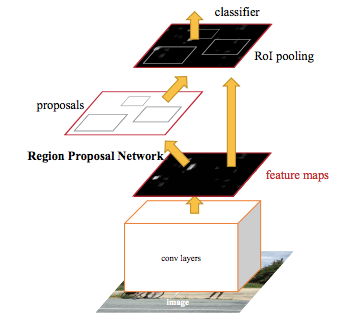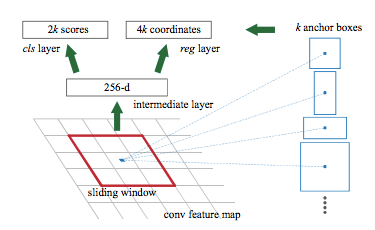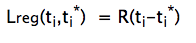# Region Proposal Network (RPN) — Backbone of Faster R-CNN

Tanay Karmarkar

#### Published on

Aug 18, 2018

• ML & AI
• Technical Deep Dive

In object detection using R-CNN, RPN is the one true backbone and have proven to be very efficient till now. Let's explore it more.

In object detection using R-CNN, RPN is the one true backbone and have proven to be very efficient till now. It's purpose is to propose multiple objects that are identifiable within a particular image.

This method was proposed by Shaoqing Ren, Kaiming He, Ross Girshick and Jian Sun in a very popular paper on "Faster R-CNN : Towards Real Time Object Detection with Region Proposal Networks". This is a very popular algorithm which attracted attention of a lot of Data Scientists, Deep Learning and AI engineers. It has enormous application like detecting objects in a self-driving car, assisting differently abled person and helping them to get independent etc.

What is CNN?

CNN translates to Convolutional Neural Networks which is a very popular algorithm for image classification and typically comprises of convolution layers, activation function layers, pooling (primarily max_pooling) layers to reduce dimensionality without losing a lot of features. For this article, you should know that there is a feature map that is generated by the last layer of convolutional layer.

For example, If you feed a cat image or a dog image, the algorithm can tell you whether it is dog or cat.

But it does not stop here, with great computational capabilities comes great advancements.

Many pre-trained models are developed to directly use them without going through the pain of training models due to computational limitation. Many models got popular as well like VGG-16, ResNet 50, DeepNet, AlexNet by ImageNet. You can find pre-trained research models from Tensorflow by google here.

For this particular article, I specifically want to talk about an algorithm or an idea which I thought was very clever from the above stated paper. Many people implement Faster R-CNN to identify the objects but this algorithm specifically dwells into the logic and math behind how algorithm gets the box around the identified objects.

The developers of the algorithm called it Region Proposal Networks abbreviated as RPN.

To generate these so called "proposals" for the region where the object lies, a small network is slide over a convolutional feature map that is the output by the last convolutional layer.Credit: Original Research Paper

Above is the architecture of Faster R-CNN. RPN generate the proposal for the objects. RPN has a specialized and unique architecture in itself. I want to further breakdown the RPN architecture.Credits: Original Research Paper

RPN has a classifier and a regressor. The authors have introduced the concept of anchors. Anchor is the central point of the sliding window. For ZF Model which was an extension of AlexNet, the dimensions are 256-d and for VGG-16, it was 512-d. Classifier determines the probability of a proposal having the target object. Regression regresses the coordinates of the proposals. For any image, scale and aspect-ratio are two important parameters. For those who don't know, aspect ratio = width of image/height of image, scale is the size of the image. The developers chose 3 scale and 3 aspect-ratio. So, total of 9 proposals are possible for each pixel, this is how the value of k is decided, K=9 for this case, k being the number of anchors. For the whole image, number of anchors is W*H*K.

This algorithm is robust against translations, therefore one of the key property of this algorithm it is translational invariant.

Presence of multi-scale anchors in the algorithm results in "Pyramid of Anchors" instead of "Pyramid of Filters" which makes it less time consuming and more cost efficient than previously proposed algorithms like Multi-Box.

But How Does It Work?

These anchors are assigned label based on two factors:

1. The anchors with highest Intersection-over-union overlap with a ground truth box.
2. The anchors with Intersection-Over-Union Overlap higher than 0.7.

Ultimately, RPN is an algorithm that needs to be trained. So we definitely have our Loss Function.Loss Function

i → Index of anchor, p → probability of being an object or not, t →vector of 4 parameterized coordinates of predicted bounding box, * represents ground truth box. L for cls represents Log Loss over two classes.p* with regression term in the loss function ensures that if and only if object is identified as yes, then only regression will count, otherwise p* will be zero, so the regression term will become zero in the loss function.

Ncls and Nreg are the normalization. Default λ is 10 by default and is done to scale classifier and regressor on the same level.

For this paper, results were obtained after training this algorithm on famous PASCAL VOC dataset.

The more advancement is being carried on in the field of instance segmentation.

If you want to go more granular, here is the link to the paper: https://arxiv.org/pdf/1506.01497.pdf.